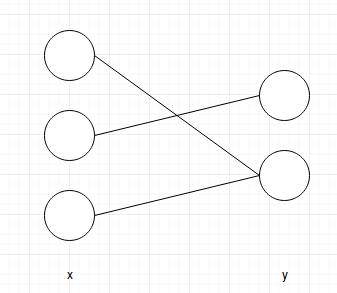# Weights disconnection implementation

I try to implement the disconnection of weights, i.e., the specific connection is always 0. It sounds like `masked_scatter_`, but I found it could not be autograded.

Here is my code:

``````import torch
import numpy as np

x = torch.rand((3, 1))
# tensor([[ 0.8525],
#         [ 0.1509],
#         [ 0.9724]])

# tensor([[ 0.3240,  0.0792,  0.6858],
#         [ 0.5248,  0.4565,  0.3625]])

# tensor([[ 0.,  1.,  0.],
#         [ 1.,  0.,  1.]])

# tensor([[ 0.0000,  0.0792,  0.0000],
#         [ 0.5248,  0.0000,  0.3625]])

# tensor([[ 0.0120],
#         [ 0.7999]])

# None

# MulBackward1 object
``````

The above code implements this graph.I want some function which is similar to `masked_scatter_` and can apply auto-gradient. Thank you in advance.

The question isn’t very clear. You to seem to be using y but it hasn’t been defined in the code above?

While I am not clear about the picture you have posted - (why is the shape of x (3,2) if there are just three input nodes). One clear issue with your code though is that none of the variables have a requires_grad=True. For autograd to track stuff at least one of the inputs should have requires_grad=True.

As far as the implementation of the diagram is concerned I would do something like this (assuming each node yields a scalar in the first layer):

``````# Layer 1
# tensor([ 1.4079,  1.5536,  0.2737])
# tensor([[ 0,  1,  0],
#         [ 1,  0,  1]])
# tensor([[ 0.0000,  1.5536,  0.0000],
#      [ 1.4079,  0.0000,  0.2737]])
# <MulBackward1 at 0x7f4b7a6725c0>
``````

If I am correct about what you are trying to do you don’t need masked_scatter but simple boolean masking.

In any case, masked_scatter_ seems to be working for me and calculating the gradients correctly.

Thank you so much for your kind help. I edited my post again. Sincere apologize for the confusion.

I am wondering under this implementation, will `mask_weights` keep tasked?

Thank you so much.

Can you clarify what do you mean by “keep tasked”?

weights.grad_fn is none because the gradients would be computed wrt weights. At the end when you finally get a scalar and call .backward the right gradients will be calculated in weights.grad. You can use an optimizer or manually update the weights from there.

I assume this is solved now?

Yes, sure, just one more little question. For the “keep tasked”, I actually refer to “disconnected”. So if I use auto back-propogation, will the disconnection still be disconnected please?

Sir, I just did an experiment, the gradients of `mask_weights` are no longer masked.

Care to share the code for your experiment

Yes, sure, thank you.

Continue my codes on the original post.

``````mask_weights.register_hook(print)

z = torch.Tensor([, ])
# tensor([[ 1.],
#         [ 1.]])

out = (y-z).mean()
# tensor(-0.6595)

out.backward()
# tensor([[ 0.1920,  0.1757,  0.0046],
#         [ 0.1920,  0.1757,  0.0046]])

As you can see, the value of gradients of `mask_weights` are not masked.